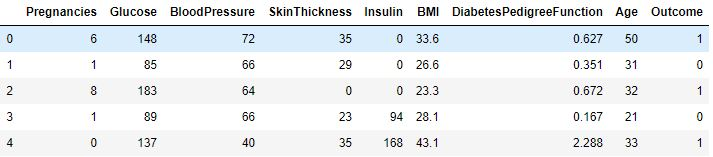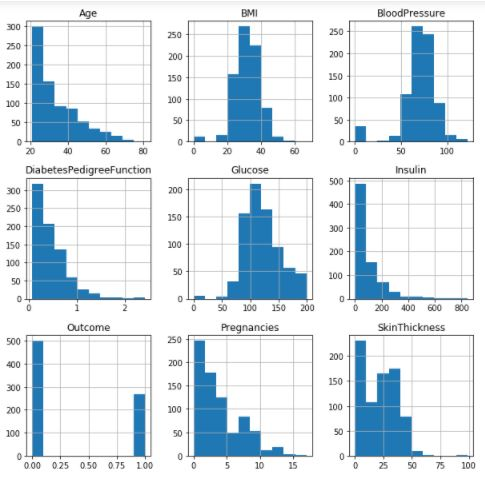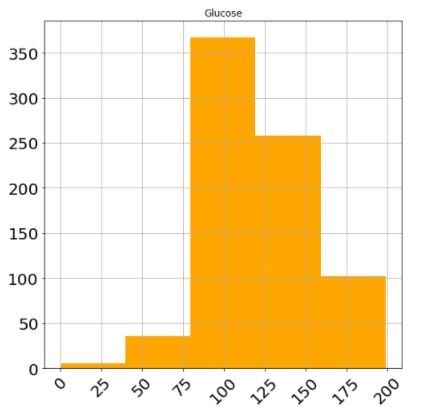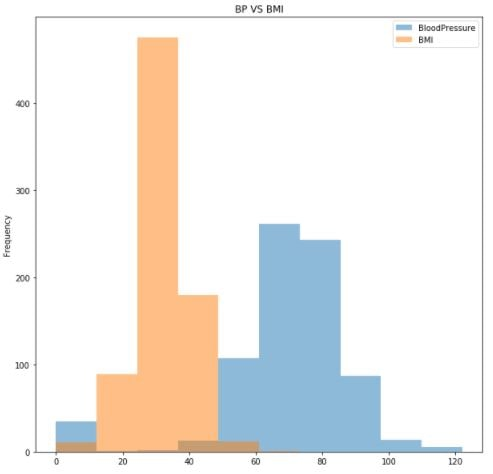In this tutorial, we will learn about how to plot Histograms using the `Pandas` library.

Pandas library provides us with a way to plot different kinds of charts out of the data frames that are easy to understand and get insights about the data. Pandas library uses the `matplotlib` as default backend which is the most popular plotting module in python.

## 1. What is a Histogram

`Histograms` are a way to visualize the distribution of the data. This further tells us about how we can perform specific operations to get better and accurate insights from the data.

## 2. Plotting Histogram in Pandas

The first step is to import the required libraries and load the data that we will be working upon. For this tutorial, we will be using the popular Pima Indians Diabetes Database. See the code below to execute these steps. All the steps are performed in Jupyter Notebooks.

``````import pandas as pd

Output:### 2.1 Plotting Histogram  of all columns

Below is the code to get the histograms of all columns of data as subplots of a single plot. We can achieve this by using the `hist()` method on a pandas data-frame. Also, We have set the total figure size as 10×10 and `bins`=10 which will divide the scale of a plot into the specified number of bins for better visualization.

``data.hist(figsize=(10,10),bins=10)``

Output:### 2.2 Plotting Histogram of a particular column and layout of plot

We have the flexibility to set some label size and rotation attributes for the plot that we are plotting which are described below.

• xlabelsize – The size of the x-axis labels in integer.
• ylabelsize – The size of the y-axis labels in integer.
• xrot – The clockwise rotation that is to be done on the labels on the x-axis.
• yrot – The clockwise rotation that is to be done on the labels on the y-axis.
• color = The colour of the plot.

See the code below to see the implementation of  attributes defined above

``````data.hist(column="Glucose", figsize=(8,8),
xlabelsize=20, ylabelsize=20,
xrot=45, bins=5, color='orange')``````

Output:### 2.3 Plotting Histograms of multiple columns in a single plot

We can also plot histograms of multiple columns inside a single plot by using the plot wrapper around the hist() method. Look at the code below to achieve this.

```data1 = data[['BloodPressure','BMI']]
ax = data1.plot.hist(alpha=0.5, figsize=(10,10),bins=10, title = "BP VS BMI")```

Output:So, We have learned how to plot histograms out of data-frames by using only the Pandas library and matplotlib as backend. If you have any doubt, feel free to ask in the comment section below.

Happy Learning 🙂